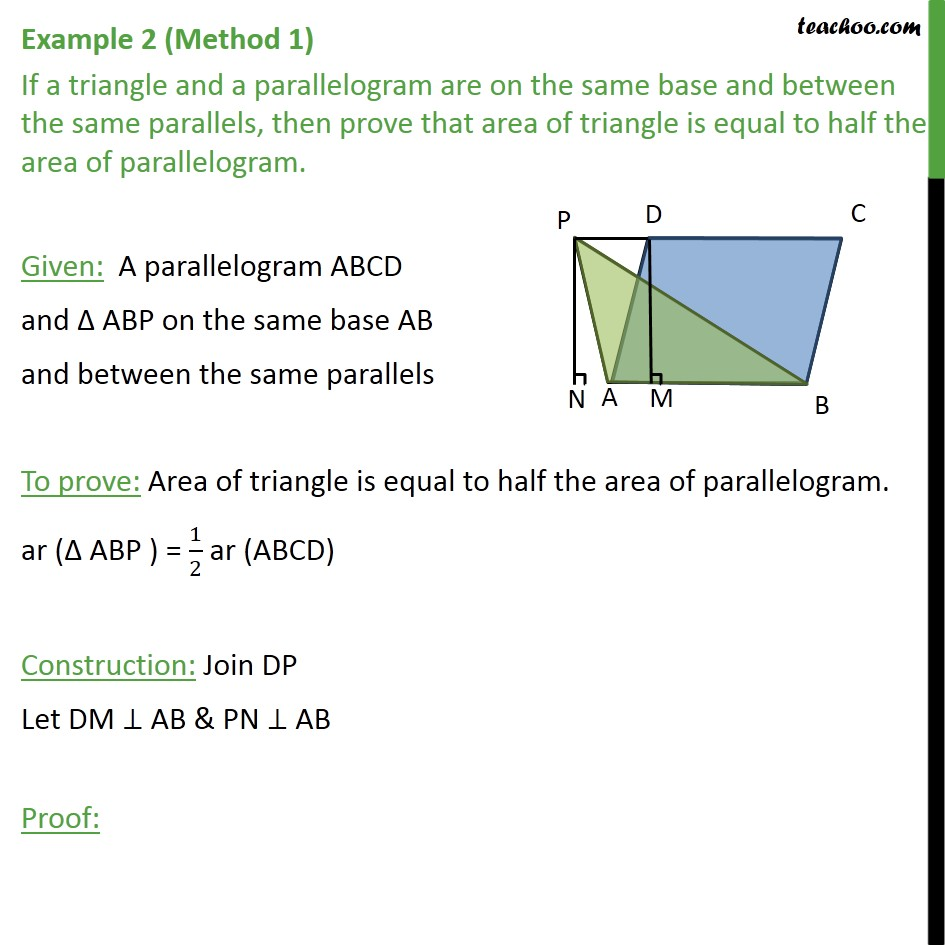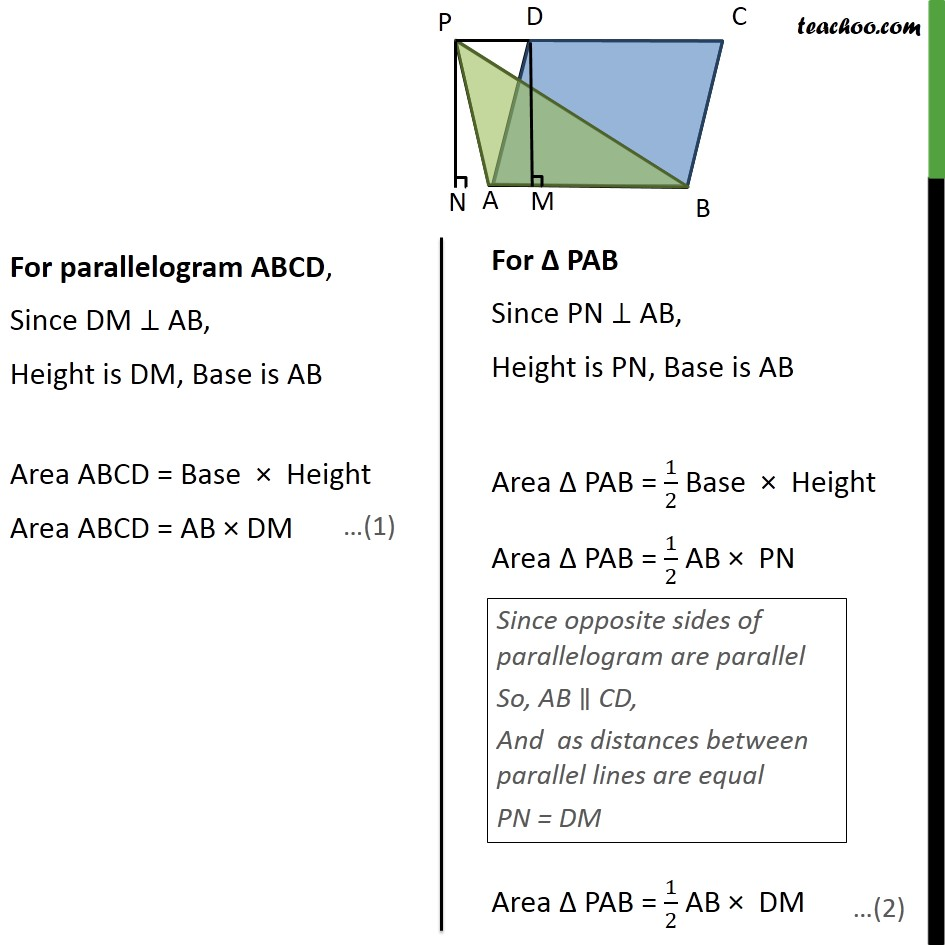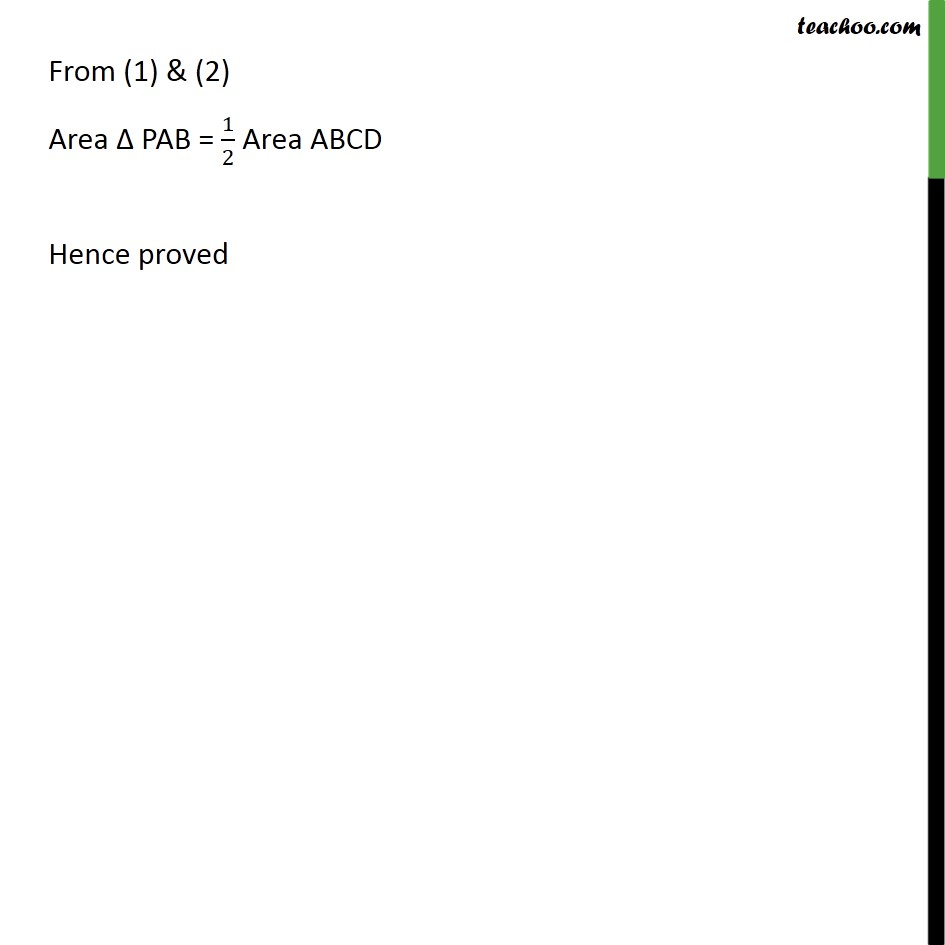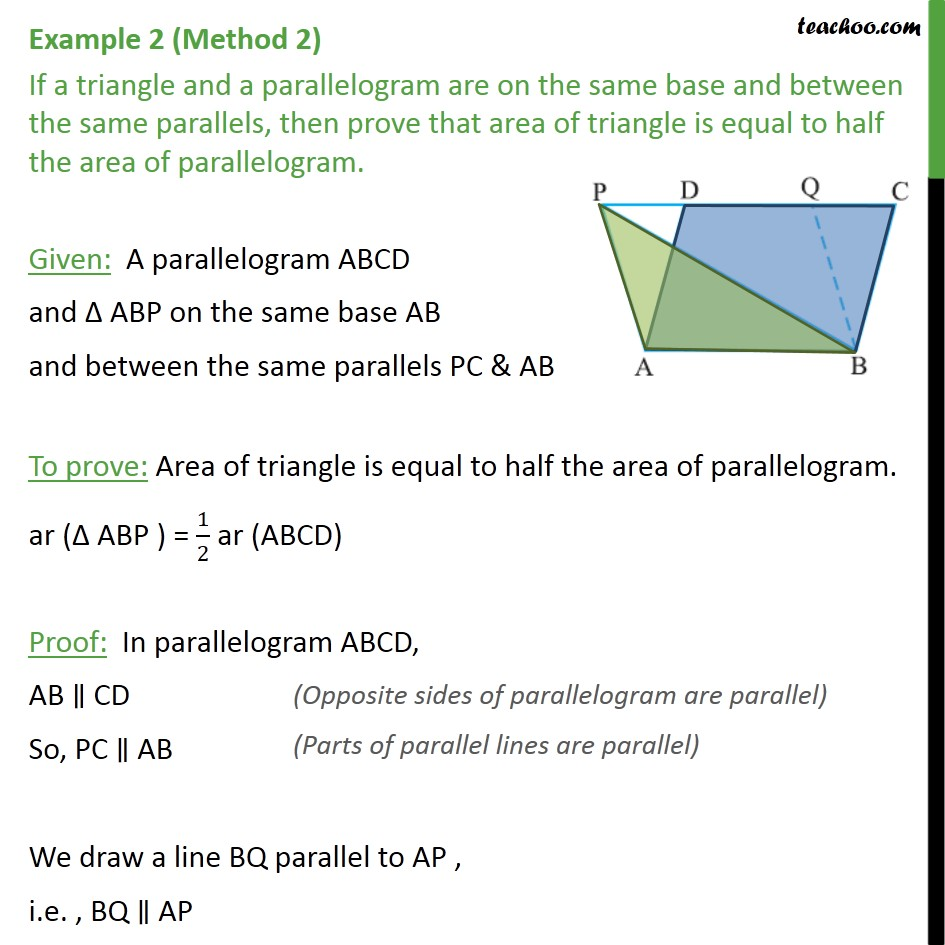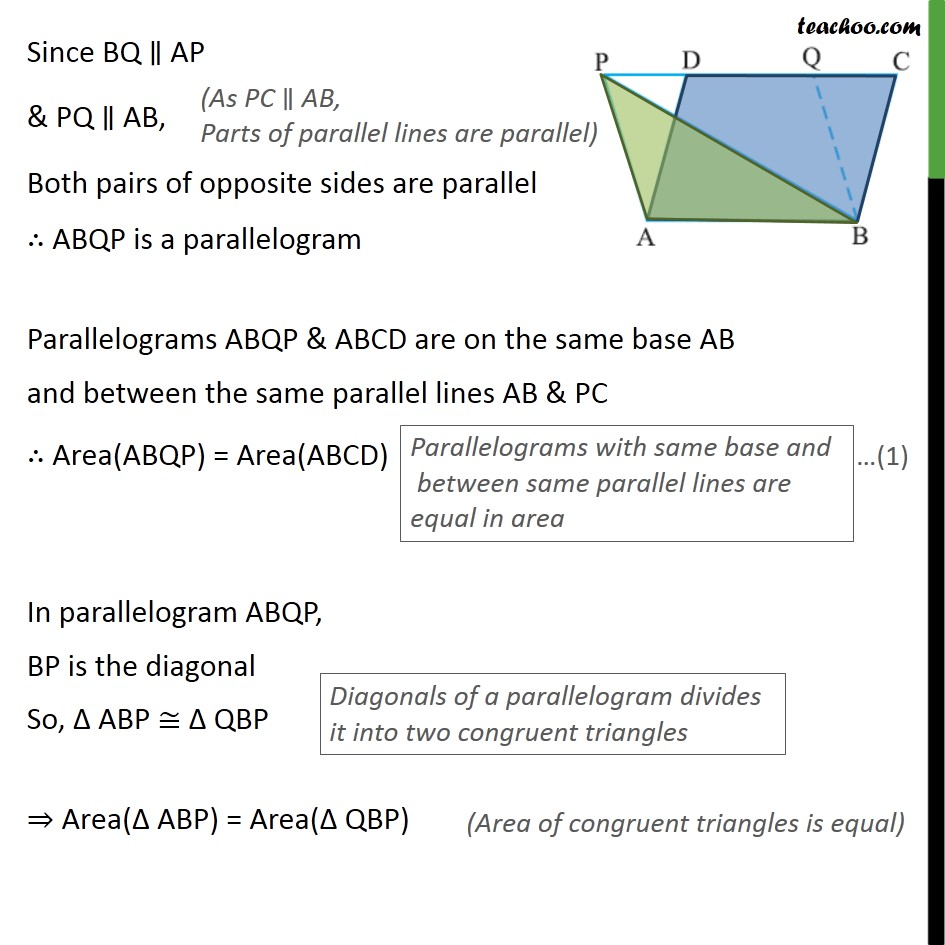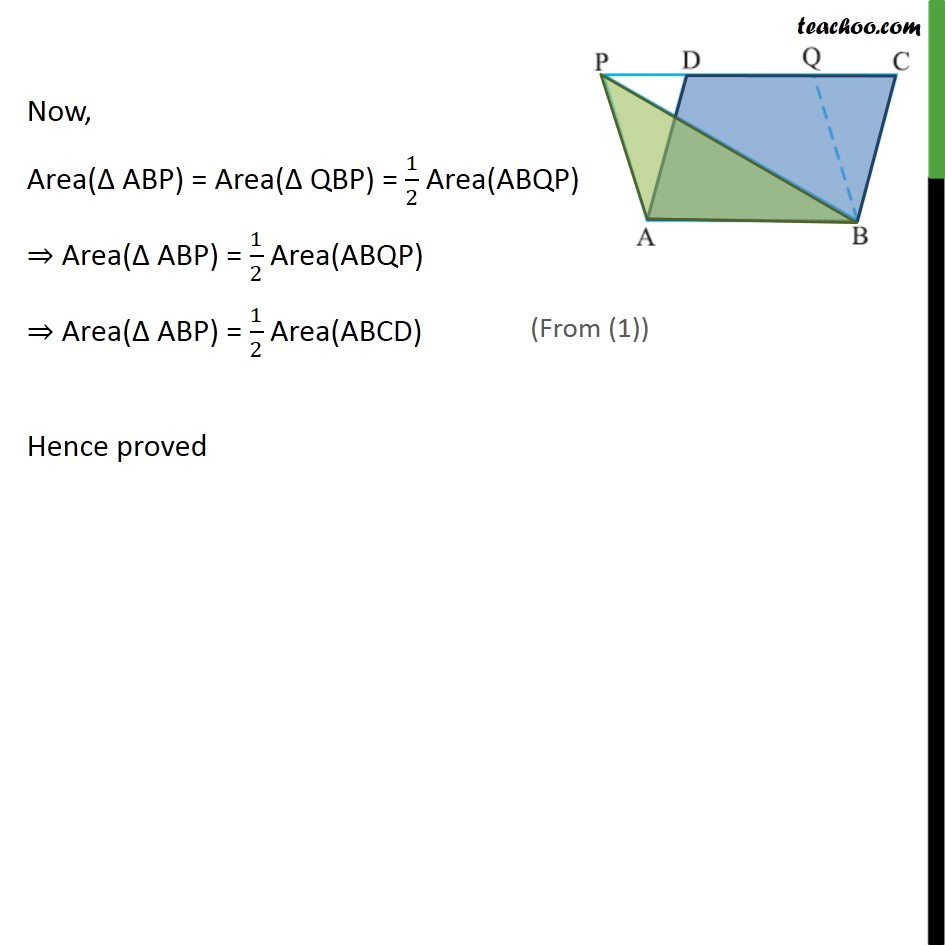Subscribe to our Youtube Channel - https://you.tube/teachoo

1. Chapter 9 Class 9 Areas of Parallelograms and Triangles
2. Concept wise
3. Paralleograms & triangles with same base & same parallel lines

Transcript

Example 2 (Method 1) If a triangle and a parallelogram are on the same base and between the same parallels, then prove that area of triangle is equal to half the area of parallelogram. Given: A parallelogram ABCD and ABP on the same base AB and between the same parallels To prove: Area of triangle is equal to half the area of parallelogram. ar ( ABP ) = 1/2 ar (ABCD) Construction: Join DP Let DM AB & PN AB Proof: Example 2 (Method 2) If a triangle and a parallelogram are on the same base and between the same parallels, then prove that area of triangle is equal to half the area of parallelogram. Given: A parallelogram ABCD and ABP on the same base AB and between the same parallels PC & AB To prove: Area of triangle is equal to half the area of parallelogram. ar ( ABP ) = 1/2 ar (ABCD) Proof: In parallelogram ABCD, AB CD So, PC AB We draw a line BQ parallel to AP , i.e. , BQ AP Since BQ AP & PQ AB, Both pairs of opposite sides are parallel ABQP is a parallelogram Parallelograms ABQP & ABCD are on the same base AB and between the same parallel lines AB & PC Area(ABQP) = Area(ABCD) In parallelogram ABQP, BP is the diagonal So, ABP QBP Area( ABP) = Area( QBP) Now, Area( ABP) = Area( QBP) = 1/2 Area(ABQP) Area( ABP) = 1/2 Area(ABQP) Area( ABP) = 1/2 Area(ABCD) Hence proved

Paralleograms & triangles with same base & same parallel lines

About the AuthorDavneet Singh
Davneet Singh is a graduate from Indian Institute of Technology, Kanpur. He has been teaching from the past 9 years. He provides courses for Maths and Science at Teachoo.